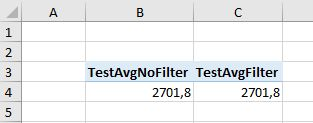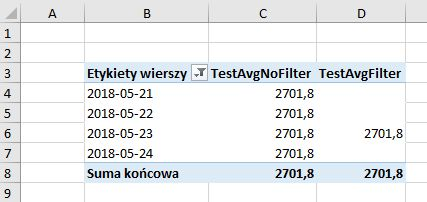2 views
in BI

What is the difference in results of CALCULATE function if we use it with and without FILTER function. Suppose we have those two measures:

Measure1 = CALCULATE([X], 'FactTable'[Color]="Red")

Measure2 = CALCULATE([X], FILTER('FactTable', 'FactTable'[Color]="Red")

Update. Additional bounty question:
Is there a way to modify Measure2 by using other functions, such as ALL, or ALLSELECTED, so that it would return exactly the same results as Measure1?

Update after bounty

I have decided to grant the bounty for a different answer than the one I accepted.

by (47.2k points)
• There is also the way of obtaining those results for both measures which will not be the same.

• I created two measures similar to your example to test this:

• TestAvgNoFilter = CALCULATE([PrcAvg]; cal[ReadDate]=DATE(2018;05;23)) TestAvgFilter = CALCULATE([PrcAvg]; filter(cal; cal[ReadDate]=DATE(2018;05;23)))

• When I simply throw both of them into pivot table without any additional fields or slicers, ofcourse both of them show the same result:• FILTER has a significant performance impact, which can be clearly seen looking at query plans and utilization of Storage Engine vs Formula Engine. It creates an additional temporary table that needs to "interact" with already existing filters coming from the report/pivot table itself (rows, columns, slicers). You won't notice anything for simple average value in a single cell, but if your [x] measure itself is complicated and there are many of those "initial" filters, the difference in calculation time can be huge.

• FILTER retains and interacts with the initial filter context, while filter expression used directly in CALCULATE ignores it. See what happens, when I add ReadDate to the pivot table:• This is precisely why the measure without FILTER is faster: it doesn't care what dates are in columns - it already calculated one "true" value, while the measure with FILTER evaluates itself against initial filters for every row.

• Results in both columns can be considered correct - it really all depends on interpretation and how you name the measures ;).

• As a general rule I would suggest you don't use FILTER when you don't have to. Save it's power for when it's really needed.